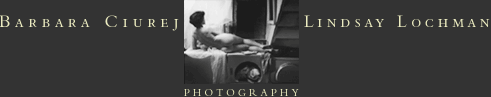M Y T H O L O G YP O R T F O L I O S :A L L   T H I N G S   A R E   A L W A Y S   C H A N G I N GT H E   C R E A T I O N   M Y T HB O M B S H E L L SG L O R Y   O N   A   B U D G E T :   A   D O M E S T I C   M Y T H O L O G YH E N P E C K E DS O M E   E N C H A N T E D   E V E N I N GF L I N G S   &   E R O SM A N   O’   T H E   W O R L DS T I L L   W ET   F R O M   T H E   C O C O O Nhome  l  artists’ statement  l  contact us